# CBSE Class 10 Science Magnetic Effects of Electric Current Assignment Set B

Read and download free pdf of CBSE Class 10 Science Magnetic Effects of Electric Current Assignment Set B. Get printable school Assignments for Class 10 Science. Standard 10 students should practise questions and answers given here for Science in Grade 10 which will help them to strengthen their understanding of all important topics. Students should also download free pdf of Printable Worksheets for Class 10 Science prepared as per the latest books and syllabus issued by NCERT, CBSE, KVS and do problems daily to score better marks in tests and examinations

## Magnetic Effects Of Electric Current Class 10 Science Assignment Pdf

Class 10 Science students should refer to the following printable assignment in Pdf for Magnetic Effects Of Electric Current in standard 10. This test paper with questions and answers for Grade 10 Science will be very useful for exams and help you to score good marks

### Class 10 Science Assignment for Magnetic Effects Of Electric Current

Question : A rectangular coil of copper wires is rotated in a magnetic field. The direction of the induced current changes once in each:
a) two revolution
b) one revolution
c) half revolution
d) one-fourth revolution

Question : At the time of short circuit, the current in the circuit
a) reduces substantially
b) does not change
c) increases heavily
d) varies continuously

Question : The magnetic field inside a long straight solenoid-carrying current
a) is zero
b) decreases as we move towards its end
c) increases as we move towards its end
d) is the same at all points

Question : At the time of short circuit, the current in the circuit
a) vary continuously
b) reduced considerably
c) does not change
d) increases heavily

Question : Match the following with correct response.a) 1-A, 2-C, 3-B, 4-D
b) 1-C, 2-B, 3-D, 4-A
c) 1-B, 2-D, 3-A, 4-C
d) 1-D, 2-A, 3-C, 4-B

Question. For a current in a long straight solenoid N- and S-poles are created at the two ends. Among the following statements, the incorrect statement is :
(A) The field lines inside the solenoid are in the form of straight lines which indicates that the magnetic field is the same at all points inside the solenoid.
(B) The strong magnetic field produced inside the solenoid can be used to magnetise a piece of magnetic material like soft iron, when placed inside the coil.
(C) The pattern of the magnetic field associated with the solenoid is different from the pattern of the magnetic field around a bar magnet.
(D) The N and S-poles exchange position when the direction of current through the solenoid is reversed.

Question. A positively-charged particle (alpha - particle projected towards west is deflected towards north by a magnetic field. The direction of magnetic field is
(A) Towards south.
(B) Towards east.
(C) Downward.
(D) Upward.

Question. A constant current flowing in a horizontal wire in the plane of the paper from East to West is shown in Figure. The direction of magnetic field at a point will be from North to South :(A) Directly above the wire.
(B) Directly below the wire.
(C) At a point located in the plane of the paper, on the north side of the wire.
(D) At a point located in the plane of the paper, on the south side of the wire.

Question. The strength of magnetic field inside a long current carrying straight solenoid is :
(A) More at the ends than at the centre
(B) Minimum in the middle
(C) Same at all points
(D) Found to increase from one end to the other

Question. Choose the incorrect statement from the following regarding magnetic lines of field :
(A) The direction of magnetic field at a point is taken to be the direction in which the north pole of a magnetic compass needle points.
(B) Magnetic field lines are closed curves.
(C) If magnetic field lines are parallel and equidistant, they represent zero field strength.
(D) Relative strength of magnetic field is shown by the degree of closeness of the field lines.

Question. Choose the correct option.
A rectangular coil of copper wires is rotated in a magnetic field. The direction of the induced current changes once in each:
(A) Two revolutions.
(B) One revolution.
(C) Half revolution.
(D) One-fourth revolution.

Question. If the key in the arrangement in the given figure is taken out (the circuit is made open) and magnetic field lines are drawn over the horizontal plane ABCD, the lines are :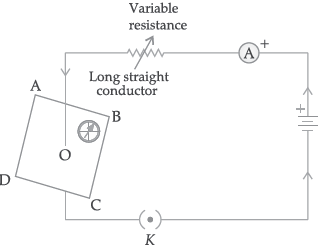(A) Concentric circles.
(B) Elliptical in shape.
(C) Straight lines parallel to each other.
(D) Concentric circles near the point O but of elliptical shapes as we go away from it.

Question. The phenomenon of electromagnetic induction is :
(A) The process of charging a body.
(B) The process of generating magnetic field due to a current passing through a coil.
(C) Producing induced current in a coil due to relative motion between a magnet and the coil.
(D) The process of rotating a coil of an electric motor.

Question. Which of the following correctly describes the magnetic field near a long straight current carrying wire?
(A) The field consists of straight lines perpendicular to the wire.
(B) The field consists of straight lines parallel to the wire.
(C) The field consists of radial lines originating from the wire.
(D) The field consists of concentric circles centered on the wire.

Question. Which of the following property of a proton can change while it moves freely in a magnetic field?
(There may be more than one correct answer.)
(A) Mass
(B) Speed
(C) Velocity
(D) Momentum

Assertion and Reason Based MCQs :

Directions : In the following questions, A statement of Assertion (A) is followed by a statement of Reason (R). Mark the correct choice as.
(A) Both A and R are true and R is the correct explanation of A.
(B) Both A and R are true but R is NOT the correct explanation of A.
(C) A is true but R is false.
(D) A is false and R is true.

Question. Assertion (A): Magnetic field lines never intersect.
Reason (R): At a particular point magnetic field has only one direction.

Question. Assertion (A): In Fleming’s Left Hand Rule, the direction of magnetic field, force and current are mutually perpendicular.
Reason (R): Fleming’s Left hand Rule is applied to measure the induced current.

Question. Assertion (A): Two bar magnets attract when they are brought near to each other with the same pole.
Reason (R): Unlike poles will attract each other.

Question. Assertion (A): An alpha particle placed in a magnetic field will not experience any force, if it moves in the magnetic field parallel to field lines.
Reason (R): The force is zero if current and field are in the same direction.

Case-based MCQs :

Read the following passage and answer the four questions given below :

A solenoid is a long helical coil of wire through which a current is run in order to create a magnetic field. The magnetic field of the solenoid is the superposition of the fields due to the current through each coil. It is nearly uniform inside the solenoid and close to zero outside and is similar to the field of a bar magnet having a north pole at one end and a south pole at the other depending upon the direction of current flow. The magnetic field produced in the solenoid is dependent on a few factors such as, the current in the coil, number of turns per unit length etc. The following graph is obtained by a researcher while doing an experiment to see the variation of the magnetic field with respect to the current in the solenoid.
The unit of magnetic field as given in the graph attached is in mili-Tesla (mT) and the current is given in Ampere.Question. What will happen if a soft iron bar is placed inside the solenoid?
(A) The bar will be electrocuted resulting in shortcircuit.
(B) The bar will be magnetised as long as there is current in the circuit.
(C) The bar will be magnetised permanently.
(D) The bar will not be affected by any means.

Question. The magnetic field lines produced inside the solenoid are similar to that of …
(A) A bar magnet
(B) A straight current carrying conductor
(C) A circular current carrying loop
(D) Electromagnet of any shape

Question. What type of energy conversion is observed in a linear solenoid?
(A) Mechanical to Magnetic
(B) Electrical to Magnetic
(C) Electrical to Mechanical
(D) Magnetic to Mechanical

Question. After analysing the graph a student writes the following statements.
I The magnetic field produced by the solenoid is inversely proportional to the current.
II The magnetic field produced by the solenoid is directly proportional to the current.
III The magnetic field produced by the solenoid is directly proportional to square of the current.
IV The magnetic field produced by the solenoid is independent of the current.
Choose from the following which of the following would be the correct statement(s).
(A) Only IV
(B) I and III and IV
(C) I and II
(D) Only II

Study the given diagram and answer the four questions given below :

In the given diagram, two coils of insulated copper wire are wound over a nonconducting cylinder as shown. Coil P has larger number of turns than coil S.Question. In above phenomenon, the current is induced in another conductor,
(A) By changing magnetic field
(B) By increasing the strength of current
(C) By decreasing the strength of the current
(D) By using extra wire.

Question. A momentary deflection is shown by the galvanometer , when:
(A) Key K is open
(B) Key K is closed
(C) In both the situations
(D) In neither of the case.

Question. Which phenomenon is involved in it ?
(A) Electromagnetic induction
(B) Magnetism
(C) Electromagnetism
(D) None of these

Question. The rule which helps us to know direction of induced current:
(A) Fleming’s right hand rule
(B) Fleming’s left hand rule
(C) Electro magnetic induction

A student fixes a sheet of white paper on a drawing board. He places a bar magnet in the centre of it. He sprinkles some iron filings uniformly around the bar magnet. Then he taps the board gently and observes that the iron filings arrange themselves in a particular pattern.

Question. What do the lines along which the iron fillings align represent ?
(A) North pole and south pole of the magnet
(B) Strength of the magnet
(C) Magnetic field lines
(D) Gravitational force.

Question. What does the crowding of iron filings at the end of the magnet indicate?
(A) Magnetic field is strongest near the poles of the magnet.
(B) Magnetic field is weakest near the poles of the magnet.
(C) There is no significant magnetic field at the poles of the magnet.
(D) The significance of polarity

Question. Why do the iron fillings arrange themselves in a particular pattern ?
(A) Due to external force applied on the magnet.
(B) Due to force exerted by the magnet outside the magnetic field.
(C) Due to the force exerted by magnet within its magnetic field.
(D) Due to pressure of magnetic field.

Question. What is SI unit of magnetic field:
(A) Pascal
(B) Nm2
(C) Tesla
(D) No unit

### Very Short Answers Type Questions :

Question : Why does the bulk of iron fillings stick to the ends of a bar magnet and not at its centre?
Answer :  Because at the ends magnetic strength is maximum and at centres magnetic strength is least.

Question : A constant current flows in a horizontal wire in the plane of the paper from East to West as shown in the figure. At what point, the direction of the magnetic field will be North to South?Answer : According to the right-hand thumb rule, the curve of the fingers represents the direction of the magnetic field. If the wire is grasped in the right hand then the direction of magnetic field will be from North to South.

Question : Which is the most important safety method used for protecting home appliances from short-circuiting or overloading?

Question : If the frequency of A.C. is 50 Hz. Then how many times it is changing its direction in 1 second?

Question : What is the pattern of the magnetic field lines around a straight conductor carrying current?

Question : If the current is flowing in the direction of advancemet of screw, then what is the direction of magnetic field lines?
Answer :   In the direction of rotation of screw.

Question :  How can you say that the magnetic field is uniform inside the solenoid.
Answer :   Because field lines are parallel inside the solenoid.

Question : Which property of a proton will change while it moves freely in a magnetic field?

Question :  According to Flemings right hand rule, which part of right hand indicate the movement of conductor?

Question :  If the no. of turns of a circular current carrying coil are doubled, then how will the magnetic field produced by it changes?

Question :  In which position the force on conductor is maximum when it is placed in uniform magnetic field?
Answer :   When conductor is Perpendicular to field

Question. Why does a compass needle show deflection when brought near a current carrying conductor ?
Answer : Due to production of magnetic field around the current carrying conductor.

Question. Draw the magnetic field lines around a straight current carrying conductor.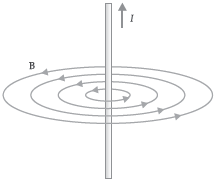Question. Why are magnetic field lines more crowded towards the pole of a magnet ?
Answer : The magnet is stronger at the poles so the magnetic field lines are crowded at the poles.

Question. The change in magnetic field lines in a coil is the cause of induced electric current in it. Name the underlying phenomenon.

Question. What is meant by magnetic field ?
Answer : The region surrounding a magnet in which the force of the magnet can be detected is said to be its magnetic field.

### Short Answers Type Questions :

Question : Diagram shows the lengthwise section of a current carrying solenoid.Decide which end of the solenoid A or B, will behave as North pole. Give reason for your answer. Also, draw field lines inside the solenoid.
Answer :  From diagram, we can see that current is entering from A and emerging out from B.Thus, using right hand thumb rule, direction of magnetic field lines is from B to A.
Thus B represent N pole and A represent S pole, as we also know that, magnetic field lines move from North to South direction out side the solenoid.

Question : Can a freely suspended current carrying solenoid stay in any direction? Justify your answer. What will happen when the direction of current in the solenoid is reversed? Explain.
Answer :   Solenoid is a closely bound cylindrical coil of insulated metallic wire. A current carrying freely suspended solenoid behaves like a magnet and when suspended freely, it rests in north-south direction.
A current carrying solenoid behaves like a bar magnet with fixed polarities at its ends. The end of the current carrying solenoid at which the current flows anticlockwise behaves as a north pole while that end at which the direction of current is clockwise behaves as a south pole. The direction of magnetic field is always perpendicular to the direction of current flow and the magnitude of the magnetic field inside a solenoid is directly proportional to the current flowing through the solenoid.
Thus, when the current through the solenoid is reversed, the direction of magnetic field is reversed.

Question : Why don't two magnetic lines of force intersect each other?
Answer :  No, two magnetic field lines can ever intersect each other. If they do, then it would mean that at the point of intersection there are two directions of magnetic field, which is not possible.

Question. An alpha particle is placed in a magnetic field.
Will it experience any force, if:
(i) it moves in the magnetic field parallel to field lines?
(ii) it moves in the magnetic field perpendicular to field lines?
Answer : (i) No, because, the force is zero if current and field are in the same direction.
(ii) Yes, because, the force is maximum when current and magnetic field are perpendicular.

Question. Give reasons for the following:
(i) There is either a convergence or a divergence of magnetic field lines near the ends of a current carrying straight solenoid.
(ii) The current carrying solenoid when suspended freely along a particular direction.
(iii) The burnt out fuse should be replaced by another fuse of identical rating.
Answer : (i) Divergence or degree of closeness of magnetic field lines near the ends of a current carrying straight solenoid indicates a increase in the strength of the magnetic field near the ends of the solenoid.
(ii) A current carrying solenoid acts as a bar magnet. We know that a freely suspended bar magnet aligns itself in the North-South direction. So, a freely suspended current carrying solenoid also aligns itself in the North-South direction.
(iii) Burnt out fuse cannot be re-used. Also, a fuse wire works because of its lower melting point. If the fuse with larger rating is used with an appliance, the fuse wire shall not melt and hence would fail to serve the required purpose. So, new fuse of same rating should be used for electrical safety.

Question. Name, state and explain with an example the rule used to determine the direction of force experienced by a current carrying conductor placed in a uniform magnetic field.
Answer : Fleming’s Left Hand Rule: The direction of force which acts on the current carrying conductor placed in a magnetic field is given by Fleming’s left hand rule. It states that if the forefinger, thumb and middle finger of left hand are stretched mutually perpendicular and if the forefinger point along the direction of external magnetic field, middle finger indicates the direction of current, then thumb points along the direction of force acting on the conductor. Example: When an electron enters a magnetic field at right angles, the direction of force on electron is
perpendicular to the direction of magnetic field and current according to this rule.

Question. Can a freely suspended current carrying solenoid stay in any direction ? Justify your answer. What will happen when the direction of current in the solenoid is reversed ? Explain.
Answer : A current carrying solenoid behaves like a magnet. When suspended freely, it will stay in north - south direction.
On reversing current its polarity will be reversed and so it will turn at 180°.

Question. State any two factors on which the magnetic field produced by a current carrying straight conductor depends.
Mention the rule which helps to find the direction of its magnetic field.
Answer : Factors on which the magnetic field produced by a current carrying conductor depends:
(i) Strength of current passing through the conductor.
(ii) Distance of the point of measurement from the conductor.
Right Hand Thumb Rule gives the direction of magnetic field.

Question. Crosses ⊗ represent a uniform magnetic field directed into the paper. A conductor XY moves in the field toward right side. Find the direction of induced current in the conductor. Name the rule you applied. What will be the direction of current if the direction of field and the direction of motion of the conductor both are reversed ?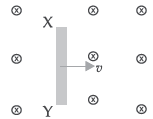Answer : (i) Y to X
(ii) Fleming’s right hand rule.
(iii) The direction of induced current will still be the same i.e., Y to X.

Question. What is meant by solenoid ? How does a current carrying solenoid behave ? Give its main use.
Answer : A coil of many circular turns of insulated copper wire wrapped closely in the shape of a cylinder is called a solenoid.
The field lines around a current-carrying solenoid is similar to that produced by a bar magnet. This means that a current carrying solenoid behaves as a magnet having north pole and south pole.
The strong magnetic field produced inside a solenoid can be used to magnetise a piece of magnetic material like soft iron when placed inside the coil.

Question. What is solenoid? Draw the field lines of the magnetic field produced on passing current through and around a current carrying solenoid.
Answer : Definition: A coil of many circular turns of insulated copper wire wrapped closely in the shape of a cylinder is called solenoid.Magnetic field lines through and around a current carrying solenoid.

### Long Answers Type Questions :

Question : State the rule to determine the direction of a (A) magnetic field produced around a straight conductor-carrying current, (B) force experienced by a current-carrying straight conductor placed in a magnetic field which is perpendicular to it, and (C) current induced in a coil due to its rotation in a magnetic field.
Answer :   Rule to determine the direction:
(A) Right hand thumb rule which states that that if one holds a straight current carrying conductor with right hand such that the thumb points towards the direction of current, then fingers will wrap around the conductor in the direction of field lines of the magnetic field.
This rule determines the magnetic field produced around a straight conductor carrying current.
(B) Fleming’s Left Hand Rule, which states that if the first finger points in the direction of magnetic field and second finger in the direction of current, then the thumb will point in the direction of motion or the force acting on the conductor.
This determines force experienced by a current-carrying straight conductor placed in a magnetic field which is perpendicular to it.
(C) Fleming’s Right Hand Rule, which states that if the forefinger indicates the direction of the magnetic field and thumb shows the direction of motion of conductor, then the middle finger will show the direction of induced current.
This rule determines the current induced in a coil due to its rotation in a magnetic field.

Question : What is the pattern of magnetic field pattern due to current carrying conductor.
Answer :  Take a straight conducting wire AB which passes through a horizontal cardboard. The ends of the wire are connected to a battery as shown in fig. When the key is closed, the current flows through the wire from B to A as shown in fig. (a), it produces magnetic field around it .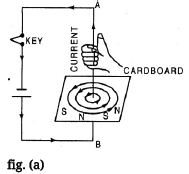.
The magnetic lines of force can be drawn with the help of a compass needle. The magnetic lines of force can also be visualized by sprinkling iron filings on the cardboard. On tapping the cardboard sheet, the iron filings arrange themselves in circles around the wire. The direction of the field is indicated by compass needle (a) The direction of magnetic field is given by right hand grip rule and by right hand cork screw rule.
Right hand grip rule is stated below : Grasp the wire in the right hand so that the thumb points along the wire in the direction of current, the fingers will then point in the direction of magnetic field.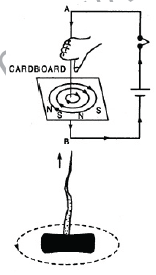Right hand cork-screw rule : Imagine a right handed cork-screw to be lying with its direction coinciding with the conductor carrying current and to be revolved so that it travels in the direction in which thumb rotates gives the direction of lines of force.

Question. What are magnetic field lines ? List three characteristics of these lines. Describe in brief an activity to study the magnetic field lines due to a current flowing in a circular coil.
(i) A rectangular cardboard having holes is used. A circular coil is passed through the holes. Coil is kept normal to the cardboard.
(ii) Ends of the coil is connected to a battery through a key.
(iii) Iron filings are sprinkled uniformly on the card board.
(iv) Key is plugged in.
(v) On tapping cardboard gently, the iron filings get arranged in concentric circular loops around the holes on the cardboard indicating the magnetic field lines.

Question. What is an electromagnet ? List any two uses.
(i) Draw a labelled diagram to show an electromagnet is made.
(ii) State the purpose of soft iron core used in making an electromagnet.
(iii) List two ways of increasing the strength of an electromagnet if the material of the electromagnet is fixed.
Answer : Electromagnet: Magnet formed by producing magnetic field inside a solenoid.
Uses of Electromagnet: Inside TVs, sound speakers and radios.
(i) Labelled diagram to show how an electromagnet is made: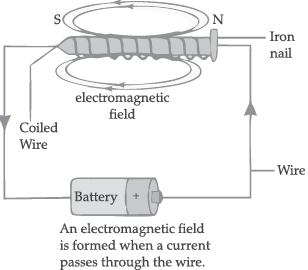(ii) Soft iron rod increases the magnetism of solenoid by a thousand fold. When the solenoid current is switched off, the magnetism is effectively switched off since the soft iron core has low retentivity.
(iii) Ways to increase the strength of an electromagnet if the material of the electromagnet is fixed are:
(a) By increasing the amount of current flowing in the solenoid.
(b) By increasing the number of turns in the solenoid.

Question. (a) What are magnetic field lines ? How is the direction of magnetic field at a point in a magnetic field determined using field lines ?
(b) Two circular coils ‘X’ and ‘Y’ are placed close to each other. If the current in the coil ‘X’ is changed, will some current be induced in the coil ‘Y’ ? Give reason.
(c) State ‘Fleming’s right hand rule”.
Answer : (a) Magnetic field line: Path along which a hypothetical free north pole would tend to move.
Direction of magnetic field are a point is determined by drawing a tangent to the magnetic field line at that point
(b) Yes.
With change in current in the coil X, the magnetic field associated with it also changes around the coil Y placed near it. This change in magnetic field induces a current in the coil Y.
(c) Fleming’s right hand rule
Stretch the thumb, forefinger and middle finger of right hand so that they are perpendicular to each other. If the forefinger indicates the direction of the magnetic field and the thumb shows the direction of motion of the conductor, then the middle finger will show the direction of induced current in the conductor.

Question. PQ is a current carrying conductor in the plane of the paper as shown in the figure below.(i) Find the directions of the magnetic fields produced by it at points R and S?
Given r1 > r2, where will the strength of the magnetic field be larger? Give reasons.
(ii) Field strength at which point will be greater?
(iii) If the polarity of the battery connected to the wire is reversed, how would the direction of the magnetic field be changed?
(iv) Explain the rule that is used to find the direction of the magnetic field for a straight current carrying conductor.
Answer : (i) The magnetic field lines produced is into the plane of the paper at position R and out of the paper at position S.
(ii) Field at S > Field at P. Magnetic field strength for a straight current carrying conductor is inversely proportional to the distance from the wire.
(iii) The current will be going from top to bottom in the wire shown and the magnetic field lines are now in the clockwise direction on the plane which is perpendicular to the wire carrying current.
(iv) Right hand thumb rule: The thumb is aligned to the direction of the current and the direction in which the fingers are wrapped around the wire will give the direction of the magnetic field.

Question. (a) Magnetic field lines of two bar magnets A and B are as shown below. Name the poles of the magnets facing each other.(b) Two magnetic field lines never intersect each other. Why ?
(c) How does the strength of the magnetic field at the centre of a current carrying circular coil depend on the:
(ii) Number of turns in the coil, and
(iii) Strength of the current flowing in the coil ?
(b) Intersection of magnetic field lines at a point means two tangents can be drawn at that point and there will be two direction of a magnetic field which is not possible.
(c) (i) Inversely proportional; more radius, less strong magnetic field.
(ii) Directly proportional; more turns, more strong magnetic field.
(iii) Directly proportional; more strength of current, more strong magnetic field.

Question. What is a solenoid ? Draw the pattern of magnetic field lines of (i) a current carrying solenoid and (ii) a bar magnet. List two distinguishing features between the two fields.
Answer : A coil of many turns of insulated copper wire wrapped closely in the shape of a cylinder.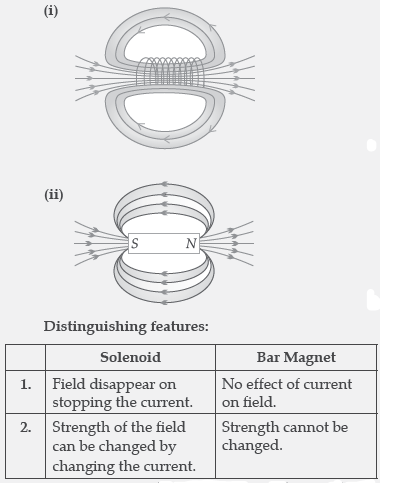Question. (i) With the help of an activity, explain the method of inducing electric current in a coil with a moving magnet. State the rule used to find the direction of electric current thus generated in the coil.
(ii) Two circular coils-I and coil-II are kept close to each other as shown in the diagram. Coil-I is connected to a battery and key and coil-II with a galvanometer. State your observation in the galvanometer:(a) When key k closed ;
(b) When key k is opened;
Give reason for you observations.
• Take two different coils of copper wire having large number of turns (say 50 and 100 turn respectively). Insert them over a nonconducting cylindrical roll.
• Connect the coil-1, having larger number of turns, in series with a battery and a plug key. Also connect the other coil-2 with a galvanometer as shown.
• Plug in the key. Observe the galvanometer. There is a deflection in its needle. You will observe that the needle of the galvanometer instantly jumps to one side and just as quickly returns to zero, indicating a momentary current in coil-II.
• Disconnect coil-I from the battery. You will observe that the needle momentarily moves, but to the opposite side. It means that now the current flows in the opposite direction in coil-II.
(ii) Same as CBSE Marking Scheme Answer.

Question. (i) Describe an activity to determine the direction of magnetic field produced by a current carrying straight conductor. Also show that the direction of the magnetic field is reversed on reversing the direction of current.
(ii) An α-particle, (which is a positively charged particle) enters a uniform magnetic field at right angles to it as shown below. Stating the relevant principle explain in which direction will this α-particle move.Answer : (i) Take a battery (12 V), a variable resistance (or a rheostat), an ammeter (0 - 5A), a plug key and a long straight thick copper wire. Insert the thick wire through the centre, normal to the plane of a rectangular cardboard. Take care that the cardboard is fixed and does not slide up or down.
Connect the copper wire vertically between the points X and Y, in series with the battery, a plug and key. Sprinkle some iron filings uniformly on the cardboard. Keep the variable of the rheostat at a fixed position. Close the key, so that current flows through the wire. Ensure that the copper wire placed between the points X and Y remains vertically straight. Gently tap the cardboard for a few times. Observe the pattern of the iron filings. You would find that the iron filings align themselves showing a pattern of concentric circles around the copper wire. This represents the magnetic field around the current-carrying conductor. The direction of magnetic field changes on reversing the direction of current.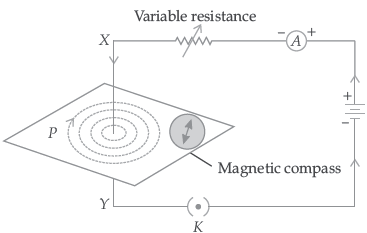(ii) The alpha particle will move in a circular path. This is because a centripetal force acts on the particle due to the movement of particle in the magnetic field.

Question. (a) State Fleming’s left hand rule.
(b) Write the principle of working of an electric motor.
(c) Explain the function of the following parts of as electric motor.
(i) Armature (ii) Brushes (iii) Split ring
Answer : (a) Fleming’s left-hand rule: Stretch the forefinger, middle finger and thumb of left hand in such a way that they are mutually perpendicular to each other. If the forefinger points in the direction of magnetic field, middle finger points in the direction of current then the thumb shows the direction of force or motion on the current carrying conductor.
(b) Principle of working of electric motor: A coil carrying electric current placed in an external magnetic field experiences a force.
(c) (i) Function of armature : It is a rectangular iron core wrapped by the copper coil through which electricity passes and due to magnetic field it experiences a force and rotates.
(ii) Function of brushes: It helps in easy transfer of charge between the coil and the external circuit.
(iii) Function of split rings: It reverses the direction of current after each half rotation of the coil so that the coil can keep rotating continuously.

Question. Draw the pattern of magnetic field lines produced around a current carrying straight conductor passing perpendicularly through a horizontal cardboard. State and apply right-hand thumb rule to mark the direction of the field lines. How will the strength of the magnetic field change when the point where magnetic field is to be determined is moved away from the straight conductor ? Give reason to justify your answer.
Answer : Pattern of magnetic field lines produced around a current carrying straight conductor:Right-hand thumb rule: If we are holding a current carrying straight conductor in right hand such that the thumb points towards the direction of current, then, the fingers will wrap around the conductor in the direction of the field lines of the magnetic field.As the compass is placed farther, deflection in the needle decreases. Thus, the magnetic field produced by given current decreases as the distance from it increases. The concentric circles around the wire become larger as we move away from it.

Question. (i) Explain with the help of the pattern of magnetic field lines the distribution of magnetic field due to a current carrying a circular loop.
(ii) Why is it that the magnetic field of a current carrying coil having n turns, is ‘n’ times as large as that produced by a single turn (loop) ?
Answer : Magnetic field due to current through a circular Loop: It can be represented by concentric circle at every point. Circles become larger and larger as we move away. Every point on wire carrying current would rise to magnetic field appearing as straight line at centre of the loop. The direction of magnetic field inside the loop is same.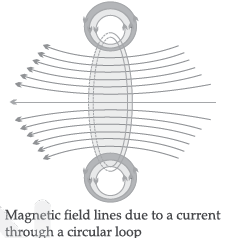Magnetic field is directly proportional to number of turns (n) in the coil. As the number of turns (n) in the coil increase, the magnetic strength at the centre increases, because the current in each circular turn is having the same direction, thus the field due to each turn adds up.

Question. (i) A coil of insulated copper wire is connected to a galvanometer. What happens if a bar magnet is:
(a) Pushed into the coil ?
(b) Withdrawn from inside the coil ?
(c) Held stationary inside the coil ? Give reasons for your observation.
(ii) Mention one more method of inducing current in a coil.
Answer : (i) (a) When a bar magnet is pushed into the coil of insulated copper wire connected to a galvanometer, an induced current is set-up in the coil due to charge of magnetic field through it. As a result, galvanometer gives a deflection (say towards left) and returns to original position.
(b) When the bar magnet is withdrawn from inside the coil, again an induced current is set up in the coil due to charge of magnetic field through it. As a result galvanometer gives a deflection in the reverse direction (say towards right) and returns to original position.
(c) If the bar magnet is held stationary inside the coil, then there is no induced current in the coil, because there is no change in magnetic field through it. As a result, galvanometer does not show any deflection.
(ii) By changing current in another coil placed near it.